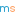# Puzzle Solutions, Issue 04

Warning: Contains SPOILERS!These solutions relate to puzzles found in Issue 04 of the magazine.

### Odd sums

The sum of the first $n$ odd numbers is $n^2$ (this can be proved by induction). This means that:

$$\frac{\text{sum of the first }n\text{ odd numbers}}{\text{sum of the next }n\text{ odd numbers}}=\frac{n^2}{(2n)^2-n^2}\\ =\frac{n^2}{3n^2}=\frac{1}{3}$$

### Odd squares

1 and 9 are the only two square numbers whose digits are all odd.

### Two lines@mscroggsmscroggs.co.uk    + More articles by Matthew